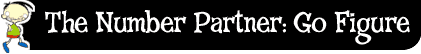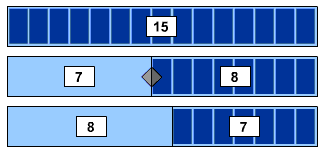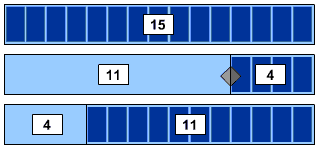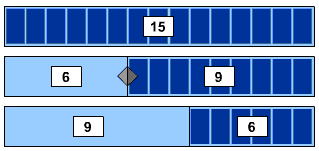﻿ The number partner: go figure - (TLF L105 v5.0.0)# Number pairs

Sometimes you come across numbers that seem really big.

Knowing how to break up a big number into two smaller numbers (a number pair) can make it easier to solve sums in your head.

Most numbers have more than one number pair.

For example, one number pair for 15 is 7 and 8.

Notice how 7 plus 8 equals 15.Another number pair for 15 is 11 and 4.

11 plus 4 equals 15.Can you think of some other number pairs for 15?

6 and 9 is another number pair for 15.

9 and 6 is the same number pair, only the numbers are around the other way.10 and 5 is another number pair for 15.

Again, 5 and 10 is the same number pair, only the numbers are around the other way.Can you think of any other number pairs for the number 15?

See if you can list all whole number pairs for 15. (Hint: there are seven different pairs).